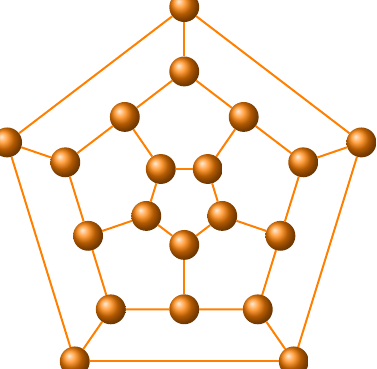# Return vertices of a Schlegel projection

I would like to have the vertices of a Schlegel's projection of a dodecahedron, octahedron, and an icosahedron, to plot them using tikz in a LaTeX document. Can I generate a tikz plot using SAGE?

Thanks.

edit retag close merge delete

Sort by » oldest newest most votedIf this helps, you can get TikZ/tkz code from sage for the Dodecahedral graph (and similarly for the Icosahedral graph) as follows.

g=graphs.DodecahedralGraph()
g.set_latex_options(tkz_style = 'Art')
print latex(g)
# very long output


Sample output after compiling the LaTeX code:However if you just want the coordinates of the vertices, then g.get_pos() will return a dictionary containing the coordinates.

more